Unknown number 2

I think the number. When he reduces it four times, I'll get 11. What number am I thinking?

Result

x =  44

Solution:Leave us a comment of this math problem and its solution (i.e. if it is still somewhat unclear...):Be the first to comment!Next similar math problems:

1. Unknown number 22I am a two-digit number. My tens digits is 4. My ones digit is 3 more than my tens. Who am I?
2. Two numbersFind the four times smaller number for numbers 12 and 36 and then add them.
3. Number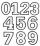Which number is 17 times larger than the number 6?
4. Written numberPlace+values x ten thousands =30 thousands
5. Bus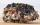On the 4-th stop take on 56 and take off 38 passengers. How many were added (write as positive number) or shrunk (write as negative number) the count of passengers?
6. ProductResult of the product of the numbers 1, 2, 3, 1, 2, 0 is:
7. Numbers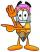By how many is the difference of numbers 8 and 34 less than its product?
8. How manzBy how many is the product of the numbers 328 and 7 greater than its sum?
9. Cakes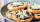On the table was 56 cakes. When Peter went home, leaving them eight times less. How many cakes left?
10. Parking field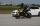There were nine cars in the parking field. The motorcycles were three times less. How many motorcycles are here and how many wheels in the parking field?
11. Fishing boat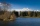The fishing boat caught 14 fish in one day. How many fish will catch 4 fishing boats in 8 days?
12. Computer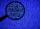A line of print on a computer contains 64 characters (letters, spacers or other chars). Find how many characters there are in 7 lines.
13. MultiplesFind all multiples of 10 that are larger than 136 and smaller than 214.
14. Curtains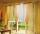For room equipment bought Hanak 50 m curtains. On the dining room needed 90 m and 90 m to hallways of curtains. How many meters of curtains bought totally?
15. 3 goods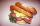Ms. Beránková bought in the shop 3 goods. Total paid for the purchase was 248 CZK. The first thing was 112 CZK and second 101 CZK. How much was the third thing?
16. Carpenter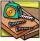You want to cut long board into smaller pieces. Carpenter said that one cut of the board into two pieces will cost you 70 cents. But you need to cut the board into 9 parts. How much you pay?
17. Rolls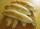Michail likes rolls, which cost 12 cents. Previously purchased 6 rolls. How many cents paid Michail for rolls?